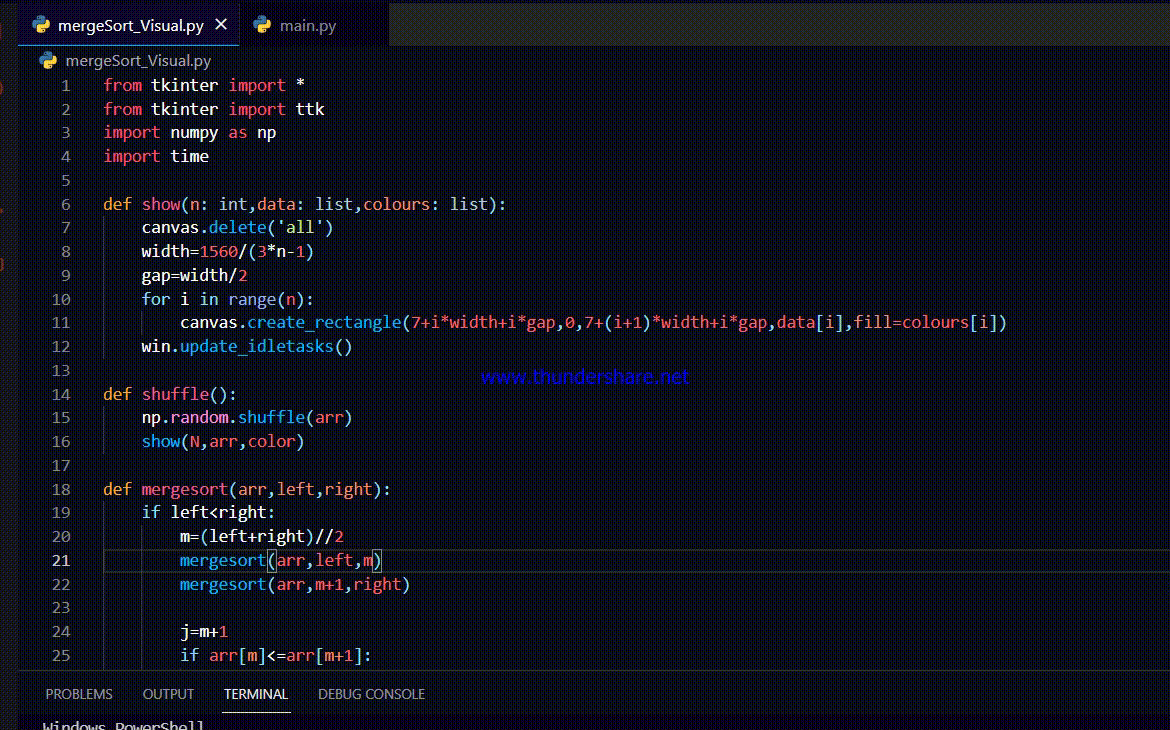Open in App
Not now

# Visualize Merge sort Using Tkinter in Python

• Last Updated : 14 Dec, 2021

Prerequisites: Python GUI – tkinter

In this article, we will create a GUI application that will help us to visualize the algorithm of merge sort using Tkinter in Python.

Merge Sort is a popular sorting algorithm. It has a time complexity of N(logN) which is faster than other sorting algorithms like a bubble or selection sort. By creating this application we will be able to visualize how exactly merge sort works. Merge sort follows the divide-and-conquer paradigm. It involves three steps

• Divide: divide the problem into a subset of similar small problems
• Conquer:  solve the subproblems recursively.
• Combine: combine the solutions of the subproblems into one to find the original answer

In merge sort, the array is divided into two nearly equal halves, and this process goes on till we reach a point where there are only two array elements to be sorted. Then we merge them such that the resultant array will be a sorted array. It helps us to understand or visualize how mergesort uses the divide and conquer technique.

## Getting Started

First, import the required modules, for the project. Import NumPy for generating the array, and shuffling it. Import Tkinter obviously to create the GUI interface. Lastly, import time to slow down the sorting process, crucial for visualization.

### Approach

• Defined a function show(), to display the array as bars. It takes three arguments n, data, and colors. N is the length of the array ‘data’. Colors is an array of colors for each and every bar to be drawn on the canvas.
• Shuffle() is a self-explanatory function, it just shuffles the array and displays it whenever the function is called.
• Then comes the real mergesort() implementation function.
• Start() function will trigger the mergesort function and then the sorting algorithm will start.
• win, a Tkinter object is created.
• Then a canvas and two buttons are created, assigned the respective functions that will be triggered upon pressing the buttons.

### Color codes:

While merging the selected two elements are shown using blue color. The red color is used if the element from the first subarray is greater than the element of the last subarray. Then it will display green once the two elements are swapped. When the array is finally sorted, the final color of all the bars will be purple.

Code:

## Python3

 `# importing all necessary modules``from` `tkinter ``import` `*``from` `tkinter ``import` `ttk``import` `numpy as np``import` `time`  `def` `show(n: ``int``, data: ``list``, colours: ``list``):` `    ``# n is length of the array data``    ``# data is the array itself``    ``# colours is an array of colors``    ``canvas.delete(``'all'``)` `    ``# width variable is the width of each bar``    ``width ``=` `1560``/``(``3``*``n``-``1``)` `    ``# gap is the spacing between the bars``    ``gap ``=` `width``/``2` `    ``for` `i ``in` `range``(n):` `        ``# this function will display an array of "bar"``        ``canvas.create_rectangle(``7``+``i``*``width``+``i``*``gap, ``0``, ``7` `+``                                ``(i``+``1``)``*``width``+``i``*``gap, data[i],``                                ``fill``=``colours[i])``    ` `    ``# this function will help us to see every step``    ``# of the sorting algorithm``    ``# the purpose of this function is to update the screen``    ``# in runtime``    ``win.update_idletasks()`  `def` `shuffle():``    ``np.random.shuffle(arr)``    ``show(N, arr, color)`  `def` `mergesort(arr, left, right):``    ``if` `left < right:``        ``m ``=` `(left``+``right)``/``/``2``        ``mergesort(arr, left, m)``        ``mergesort(arr, m``+``1``, right)` `        ``j ``=` `m``+``1``        ``if` `arr[m] <``=` `arr[m``+``1``]:``            ``return` `        ``while` `left <``=` `m ``and` `j <``=` `right:``            ``show(N, arr, [``'blue'` `if` `x ``=``=` `left ``or` `x ``=``=``                          ``j ``else` `'grey'` `for` `x ``in` `range``(N)])``            ``time.sleep(``1``/``speed)``            ``if` `arr[left] <``=` `arr[j]:``                ``left ``+``=` `1``            ``else``:``                ``show(N, arr, [``'red'` `if` `x ``=``=` `left ``or` `x ``=``=``                              ``j ``else` `'grey'` `for` `x ``in` `range``(N)])``                ` `                ``# array of colours where only the focused bars``                ``# are displayed red since left >arr[j]``                ``time.sleep(``1``/``speed)``                ``temp ``=` `arr[j]``                ` `                ``# storing the smaller element in temp variable``                ``i ``=` `j``                ``while` `i !``=` `left:``                    ``arr[i] ``=` `arr[i``-``1``]``                    ``show(N, arr, [``'red'` `if` `x ``=``=` `i ``or` `x ``=``=``                                  ``j ``else` `'grey'` `for` `x ``in` `range``(N)])``                    ``time.sleep(``1``/``speed)``                    ``i ``-``=` `1``                ` `                ``# this while loop will shift all the elements one step``                ``# to right to make the place empty for the temp variable``                ``# upon reaching the desired location i.e. left, the``                ``# temp value will be inserted into that location.``                ``# this process is much like insertion sort``                ``arr[left] ``=` `temp` `                ``show(N, arr, [``'green'` `if` `x ``=``=` `left ``or` `x ``=``=``                              ``j ``else` `'grey'` `for` `x ``in` `range``(N)])``                ``time.sleep(``1``/``speed)``                ``left ``+``=` `1``                ``m ``+``=` `1``                ``j ``+``=` `1` `# this function call the mergesort function which will``# start the animation.``def` `start():``    ``mergesort(arr, ``0``, N``-``1``)``    ``show(N, arr, [``'purple'` `for` `_ ``in` `range``(N)])`  `win ``=` `Tk()` `N ``=` `50`  `# length of the array``speed ``=` `100`  `# how fast the array will be sorted` `# creating the array using linspace function``# from numpy``arr ``=` `np.linspace(``10``, ``390``, N, dtype``=``np.uint16)` `color ``=` `[``'grey'` `for` `_ ``in` `range``(N)]` `ttk.Label(win, text``=``'Merge Sort visualizer'``).pack()``canvas ``=` `Canvas(win, width``=``800``, height``=``400``, bg``=``'white'``)``canvas.pack()` `ttk.Button(win, text``=``'Start sorting'``, command``=``start).pack(``    ``side``=``'right'``, padx``=``5``, pady``=``5``)` `ttk.Button(win, text``=``'Shuffle array'``, command``=``shuffle).pack(side``=``'right'``)``shuffle()``show(N, arr, color)` `win.mainloop()`

Output:My Personal Notes arrow_drop_up